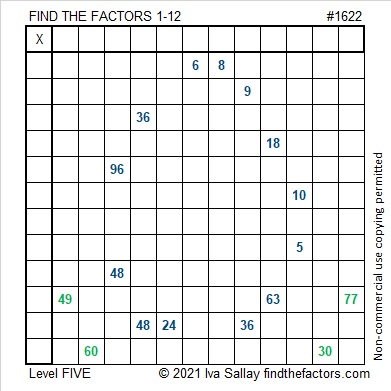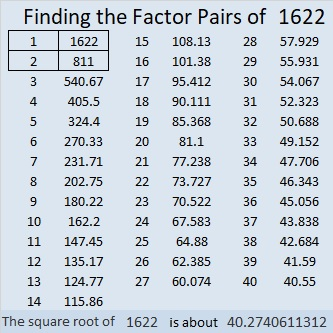# 1622 A Blue Egg for Your Easter Basket

### Today’s Puzzle:

These somewhat tricky level-5 puzzles are probably better suited for middle school and up than younger kids. Use logic on every step and you should be able to find its unique solution.### Math Eggs from Twitter:

Here are some Easter egg puzzles I saw on Twitter. Some are perfect for the littles and others are for older kids. Easter egg hunts can be fun for anyone of any age.

### Factors of 1622:

• 1622 is a composite number.
• Prime factorization: 1622 = 2 × 811.
• 1622 has no exponents greater than 1 in its prime factorization, so √1622 cannot be simplified.
• The exponents in the prime factorization are 1 and 1. Adding one to each exponent and multiplying we get (1 + 1)(1 + 1) = 2 × 2 = 4. Therefore 1622 has exactly 4 factors.
• The factors of 1622 are outlined with their factor pair partners in the graphic below.### More about the Number 1622:

1622 is the sum of four consecutive numbers:
409 + 410 + 411 + 412 = 1622.